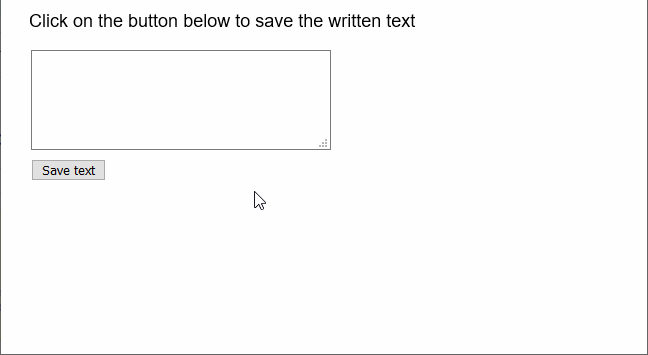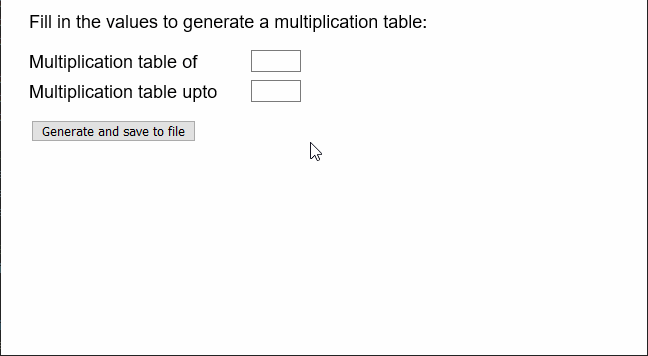GeeksforGeeks App
Open AppBrowser
Continue

# p5.js | createWriter() Function

The createWriter() function in p5.js is used to create a p5.PrintWriter object that can be used to write or print to various available streams.

Syntax:

`createWriter( name, [extension] )`

Parameters: This function accepts two parameters as mentioned above and described below.

• name: It is a string that denotes the name of the file to be created.
• extension: It is a string that specifies the extension of the file. It is an optional parameter.

Return Value: It returns a p5.PrintWriter object that denotes the writer.

The example below illustrates the createWriter() function in p5.js:

Example 1:

 `let fwriter;`` ` `function` `setup() {``  ``createCanvas(600, 300);``  ``textSize(18);`` ` `  ``// Create a textarea for the input of text``  ``inputArea = createElement(``"textarea"``);``  ``inputArea.position(30, 50);``  ``inputArea.size(300, 100);`` ` `  ``// Create a button for saving text``  ``saveBtn = createButton(``"Save text"``);``  ``saveBtn.position(30, 160);``  ``saveBtn.mousePressed(saveFile);`` ` `  ``// Setup the writer``  ``fwriter = createWriter(``"note.txt"``);`` ` `  ``text(``"Click on the button below to save the written text"``, 20, 20);``}`` ` `function` `saveFile() {``  ``// Get the value of the textarea``  ``// and split the strings on the basis``  ``// of the nextline character``  ``stringList = inputArea.value().split(``"\n"``);`` ` `  ``// Save the strings to file``  ``for` `(line of stringList) {``    ``fwriter.print(line);``  ``}`` ` `  ``// Close the writer``  ``fwriter.close();`` ` `  ``// Clear the writer``  ``fwriter.clear();``}`

Output:Example 2:

 `function` `setup() {``  ``createCanvas(600, 300);``  ``textSize(18);`` ` `  ``// Create two inputs for the``  ``// multiplication table``  ``multiOf = createInput();``  ``multiOf.position(250, 50);``  ``multiOf.size(50);`` ` `  ``multiTo = createInput();``  ``multiTo.position(250, 80);``  ``multiTo.size(50);`` ` `  ``// Create a button for saving text``  ``saveBtn = createButton(``"Generate and save to file"``);``  ``saveBtn.position(30, 120);``  ``saveBtn.mousePressed(saveFile);`` ` `  ``// Setup the writer``  ``fwriter = createWriter(``"tables.txt"``);``}`` ` `function` `draw() {``  ``clear();``  ``text(``"Fill in the values to generate a multiplication table:"``, 20, 20);``  ``text(``"Multiplication table of"``, 20, 60);``  ``text(``"Multiplication table upto"``, 20, 90);``}`` ` `function` `saveFile() {``  ``// Get the value of the two inputs``  ``// and generate table``  ``let multipicand = multiOf.value();``  ``let multiMax = multiTo.value();`` ` `  ``for` `(let multiplier = 1; multiplier <= multiMax; multiplier++) {``    ``let textToWrite =``      ``multipicand + ``" * "` `+ multiplier + ``" = "` `+ multipicand * multiplier;`` ` `    ``// Print to the writer``    ``fwriter.print(textToWrite);``  ``}`` ` `  ``// Close the writer``  ``fwriter.close();`` ` `  ``// Clear the writer``  ``fwriter.clear();``}`

Output:Online editor: https://editor.p5js.org/

My Personal Notes arrow_drop_up
Related Tutorials